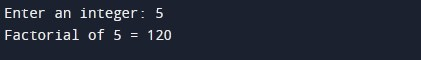C Program To find factorial of a number

In this tutorial you will learn about the C Program To find factorial of a number and its application with practical example.

In this tutorial, we will learn to create a program find factorial of a given number using C programming language. We will show you following different method to find factorial of a given number:

• factorial of a number using for loop
• factorial of a number using function
• factorial of a number using recursion

Prerequisites

Before starting with this tutorial we assume that you are best aware of the following C programming topics:

• C Data Types
• C Operator
• C If else
• C for loop
• C Function
• C Recursion

What is factorial?

Factorial of a positive integer number n is the product of all it’s positive descending integers till 1. Factorial of a positive number n is represented as following.

Mathematically !(bang) sign is used to represent factorial of given number.

Example:-

Factorial 5 is represented as following:

Similarly, factorial of 3 is represented as following:

Program To find factorial of a number using for loop

In this program we will find the factorial of a number using for loop. We have first declared and initialized the required variables. Next, we would prompt user to input an integer number. Later in the program we will find the factorial of the number using for loop. We will then display the factorial of the given number to user.

Output:-In the above program, we have first declared and initialized a set variables required in the program.

• num = it holds the first integer number value
• i = iterator for loop
• fact = it holds the factorial of the given number, initialized with 1

In the next statement user will be prompted to enter an integer number which will be assigned to variable ‘num’. Next, we will compute the factorial of the given number using c for loop. Now, we will be displaying the factorial of the given number using printf statement.

Program To find factorial of a number using function

In this program we will find the factorial of a number using c function. We have first declared and initialized the required variables. Next, we would prompt user to input an integer number. Later in the program we will find the factorial of the number using a user defined function. We will then display the factorial of the given number to user.

Output:-In the above program, we have first defined following function:

factorial() :- This function means to accept an integer number and return the factorial value of that number.

Next, we have declared and initialized a set variables required in the program.

• num = it holds the first integer number value
• i = iterator for loop
• fact = it holds the factorial of the given number, initialized with 1

In the next statement user will be prompted to enter an integer number which will be assigned to variable ‘num’. Next, we will be calling factorial() function to compute and return factorial value of the ‘num’ variable. Finally, we will be displaying the return value of the factorial() function using printf statement.

Program To find factorial of a number using recursion

In this program we will find the factorial of a number using a recursive function. A function is called recursive when calls itself. We have first defined a recursive function in the program to return factorial of the given number. Next, we have declared and initialized the required variables. We would then prompt user to input an integer number. Later in the program we have invoked the factorial function to find factorial value of the number. We will then display the factorial of the given number to user.

Output:-In the above program, we have first defined a function called as factorial() that will recursively compute the factorial of the given number. Later in the main function, we declared required variables.

• num = it holds the first integer number value

In the next statement user will be prompted to enter an integer number which will be assigned to variable ‘num’. Next, we will be calling factorial() function to compute and return factorial value of the ‘num’ variable. Finally, we will be displaying the return value of the factorial() function using printf statement.

In this tutorial we have learn about the C Program To find factorial of a number and its application with practical example. I hope you will like this tutorial.